## The Sample Mean and Its Mean and Standard Deviation

Our task here is to look at the mean and standard deviation of samples in general. We start with some original population that has a known mean, μ, and standard deviation, σ. We take repeated samples, each of size n, and for each sample we generate a sample mean,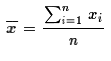, (and, although we will not make much use of it, the sample standard deviation,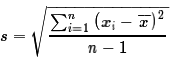). Then, we want to treat the collection of sample means as a population itself and we want to examine the mean and standard deviation of that population. Figure 1 attempts to show this.

Figure 1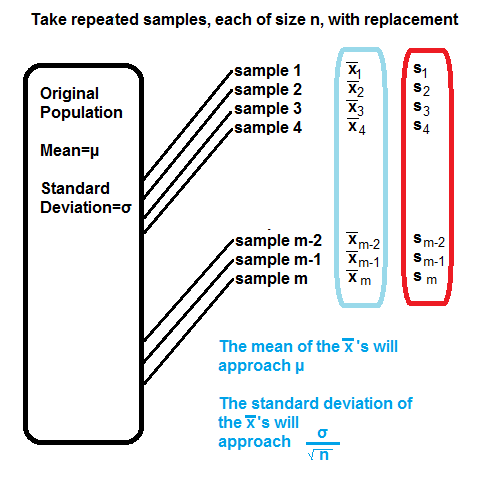As suggested in Figure 1, the mean of all the sample mean values should be really close to the mean of the original population. Furthermore, the standard deviation of the sample means should be really close to the value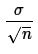.

It has been my experience that this concept needs a great deal of justification in terms of taking actual populations and then actual repeated samples. The rest of this page will walk you through just one example. You will be well served by repeating this process many times, perhaps altering parameters as you go, and examining the outcome each time.

We have a web page to set up the population, determine the mean and standard deviation of the original population, and determine the size of our repeated samples. A small image of that page is shown in Figure 2.

Figure 2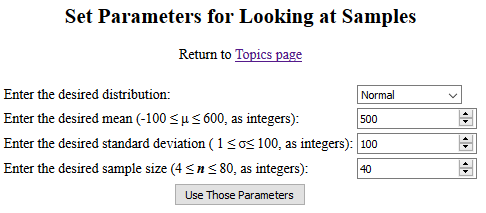This first time going to that page we want to leave the values as given and just click on the "Use Those Parameters" button. Doing so will take you to a new page that will have the population, information about the population, 1000 different samples taken from the population, and information about the population of the sample means.
 It is important to note that each time you go to this subsequent page, or each time you refresh(reload) it, the new page will generate all new values for its population and it will take all new samples from that population. As such, the images shown below will have different values on them than the values you will see when you go to the page.

The new page is far too long to reproduce here. I did manage to get a pdf version of it and, if you wanted to, you could look in a new tab at the page that was generated by clicking on the link: takesamples01.pdf. The page does have some major sections to it however, and we will look at some portion of each section.

The page starts by confirming the kind of population requested along with the desired population mean and standard deviation. Then the page displays the population of 1000 values that has been created according to those specifications. In Figure 3 we see the top of the new page. (You might note that the first item in the population is indexed as item 0 rather than as item 1.)

Figure 3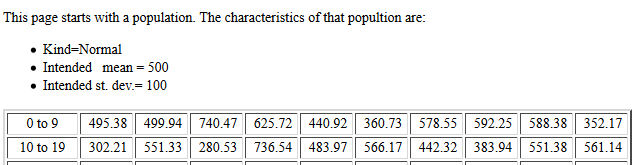At the end of the listing of the items, the web page displays the actual mean and standard deviation of the population, just so that we can compare it to the desired values given in Figure 3. Then the page goes on to list the 1000 values again, but this time in sorted order, from the smallest to the largest value. Figure 4 shows that the population mean and standard deviation are exactly as we had requested. (At times the web page may be off by a little, but that will be a very small difference.)

Figure 4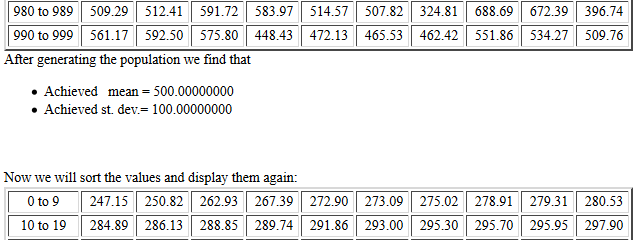At the bottom of Figure 4 we see the start of the sorted list of values. One consequence of this is that we now know the minimum value in the population. It is 247.15.

We continue to read down the list until we reach the end, shown in Figure 5, where we see that the maximum value in the population is 825.22.

Figure 5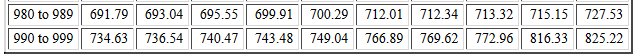After the sorted listing of the population values the web page displays a histogram of the values. The histogram should have about 26 intervals. The intervals are labelled on the graph and the details of the intervals are given in a table below the graph. For example, in our histogram, shown in Figure 6, interval "L" represents the frequency of values between 500 and 525, including 500 but not 525. The chart shows that there were 94 such values in our population.

Figure 6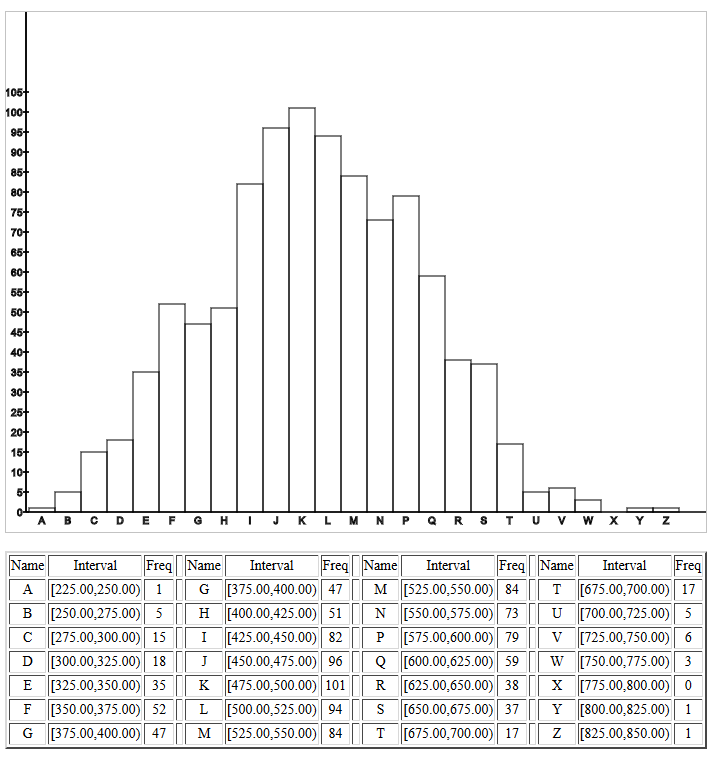Recall that we had asked for a normal population. The histogram in Figure 6 fits that description.

Below that nice looking histogram is another one, produced in the old style that we used to use when output devices were line printers or non-graphic terminals. Figure 7 shows the start of this histogram. The histogram is produced sideways. Because we have much more room for this histogram, we can have many more intervals.

Figure 7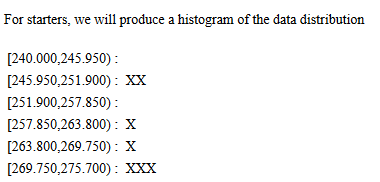The "line printer" histogram also resembles a normal distribution. It is just one more confirmation that the population is as we desired.

After that histogram, the page goes on to display the mean and standard deviation of each of 1000 samples each of size 40. Again, the information is provided just so that we can see it if we need to. It is certainly worth a bit of time to look at the values in this table. Just looking at the values shown in Figure 8 for the first 20 samples we see that the sample mean can change quite a bit, from 468.62 to 510.44 in those twenty items. However, the sample mean does not stray all that far from the population mean which we recall was 500. And, just as an observation, though we really will not use it, the sample standard deviations are not all that far away from the value of 100, the population standard deviation.

Figure 8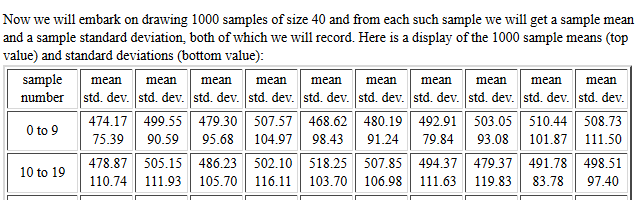After displaying the table of sample means and standard deviations, the page presents an important summary. Let us consider what the information in Figure 9 is telling us.

Figure 9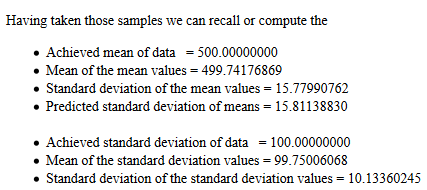• We already knew that the population had a mean of 500.
• The mean of the 1000 sample means is 499.74176869. This conforms to the much earlier statement that the mean of the sample means will approach the population mean.
• The standard deviation of the sample means is 15.77990762.
• The much earlier statement was that the standard deviation of the sample means would approach being normal with standard deviation =. The population standard deviation was essentially 100. The samples were of size 40. The value of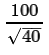is about 15.8113883. This is the value expressed as the "predicted value." Thus, the actual standard deviation of the sample means approaches.
• We already knew that the population had a standard deviation of 100.
• We can see that the mean of the sample standard deviations is 99.75, essentially the same value.
• The standard deviation of the 1000 sample standard deviations was 10.1336. Assuming that the sample standard deviations are normally distributed that means that over 95% of the values in the table should be between 79 and 121.
The web page then continues by showing us a histogram of the 1000 sample mean values. This is shown in Figure 10.

Figure 10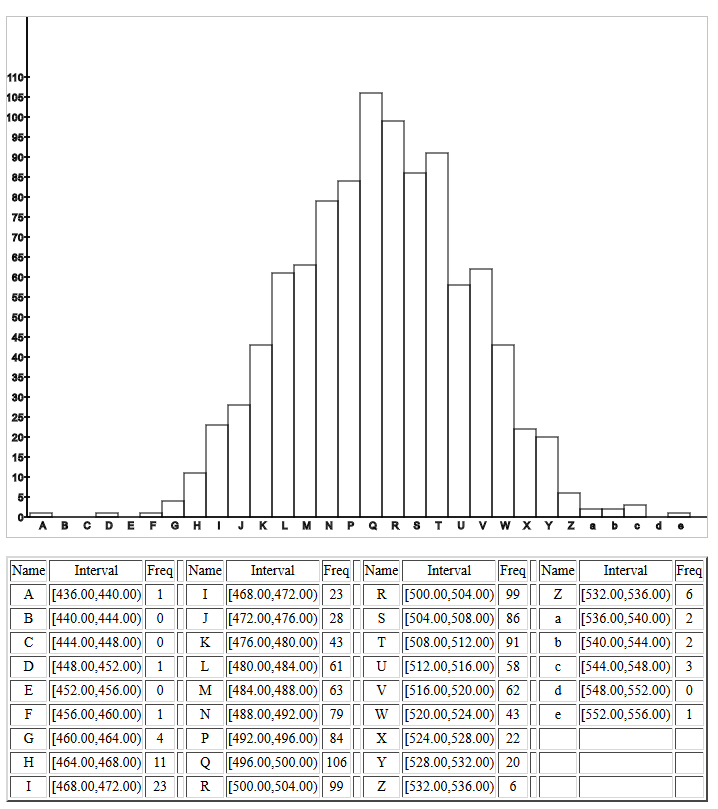The earlier assertion was that the sample means, the 1000 sample means that the web page took, would be a normal distribution. The histogram in Figure 10 certainly fits that description.

Following the pretty histogram we are given the lowest and highest sample mean values. That is followed by another "line printer" histogram, this time of the sample means.

Figure 11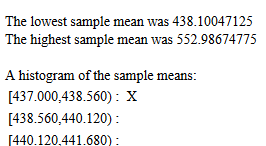Figure 12 shows a small version of the middle of the "line printer" histogram, rotated to make it easier to read, giving us another confirmation that the sample means are normally distributed.

Figure 12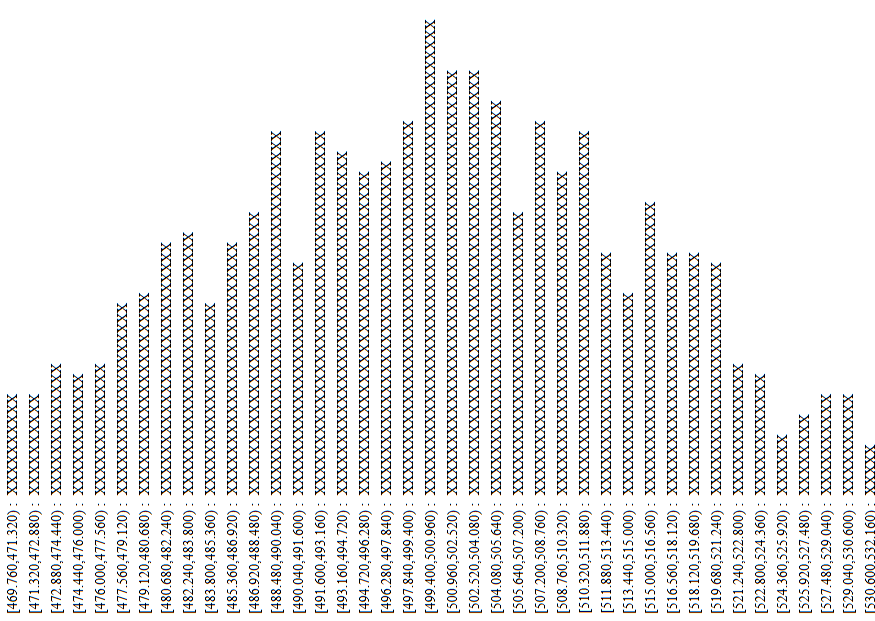The web page that we have just "walked through" in Figures 3 through 12, started with a population that was N(500,100) and ended with a population of sample means that was N(500, 100/sqrt(40)). The fact is that you could return to the web page shown in Figure 2, change the initial parameters, including starting with a distribution that is not normal, and the sample means will approach having a normal distribution with mean=μ and standard deviation=σ/sqrt(n) where μ is the population mean, σ is the population standard deviation, and n is the size of the samples.

That fact, that the sample means will approach being N( μ, σ/sqrt(n) ) is central to the rest of this course. We will be using it over and over. Therefore, it is well worth spending some time starting at the web page in Figure 2, choosing different parameters, clicking the button to move to the new page, and then reading through that page, as we did in Figures 3 through 12, to confirm our statement.

There is one additional caution on this. If you start with a normal distribution then just about any size for the samples will work. If you start with a distribution that is not normal then the "normality" of the sample means becomes more and more questionable as the size of the sample drops below 30. In all cases, the larger the sample size the more the sample means will conform to our expectations.